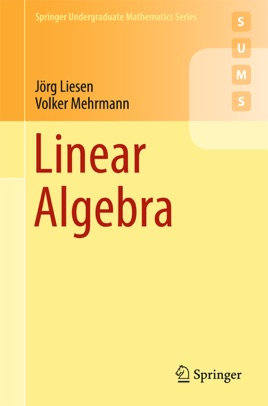• 28,99 €

## Description de l’éditeur

This self-contained textbook takes a matrix-oriented approach to linear algebra and presents a complete theory, including all details and proofs, culminating in the Jordan canonical form and its proof. Throughout the development, the applicability of the results is highlighted. Additionally, the book presents special topics from applied linear algebra including matrix functions, the singular value decomposition, the Kronecker product and linear matrix equations.
The matrix-oriented approach to linear algebra leads to a better intuition and a deeper understanding of the abstract concepts, and therefore simplifies their use in real world applications. Some of these applications are presented in detailed examples. In several ‘MATLAB-Minutes’ students can comprehend the concepts and results using computational experiments. Necessary basics for the use of MATLAB are presented in a short introduction. Students can also actively work with the material and practice their mathematical skills in more than 300 exercises.